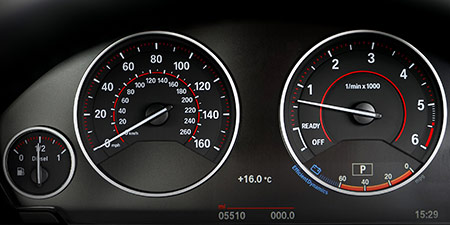# Miles per hour (mph) to Yards per hour (yd/h)

Units of speed ﹣ Converter Miles per hour to Yards per hour

Here you can convert the Speed unit Miles per hour into the unit Yards per hour and vice versa you can convert Yards per hour into Miles per hour. By clicking the "Swap units" icon, you will always obtain the desired conversion in the calculation result, i.e., mph to yd/h or yd/h to mph. With the following calculator you can also calculate any other Speed unit.

## Info about "Miles per hour"

The unit of length known as a "mile" and it should not be confused with the nautical mile and very commonly used in the Anglo-American countries. Therefore, the metric system is defined in miles (instead of kilometers) per time unit. For example, if a vehicle travels at a speed of 100 miles per hour (100 mph), it will move around 160 km per hour in the metric system.

The units are defined as follows: An object moving at 1 "mph" per hour will cover a distance of one mile. Therefore, 1 mile is equivalent to 1.609344 kilometers.

## Info about "Yards per hour"

The length unit "Yard" is commonly used in the Anglo-American countries. For example, speeds are represented by yard per unit of time.

The unit is defined as follows: An object moving at 1 "Yard per hour" per hour will cover a distance of one yard. Therefore, 1 yard is equivalent to 0.9144 meters (3 feet).

## Basis for conversion Miles per hour (mph) to Yards per hour (yd/h) and vice versa

The abbreviation for the "Speed unit Miles per hour" is mph. The abbreviation for the "Speed unit Yards per hour" is yd/h.

## Formula for the conversion of Miles per hour (mph) to Yards per hour (yd/h) and vice versa

The calculation from Miles per hour to Yards per hour shall be made using the following conversion formula:

Conversion formula Miles per hour to Yards per hour

Determine the number of Yards per hour from Miles per hour

Miles per hour × 1760

## Formula for the conversion of Yards per hour (yd/h) to Miles per hour (mph)

The calculation from Yards per hour to Miles per hour shall be made using the following conversion formula:

Conversion formula Yards per hour to Miles per hour

Determine the number of Miles per hour from Yards per hour

Yards per hour × 0.00056818181841042

## Overview table : How many Miles per hour are in a Yards per hour ?

Miles per hour mph ⇒ Yards per hour yd/h
0.01 mph  are  17.60000 yd/h
0.02 mph  are  35.20000 yd/h
0.03 mph  are  52.80000 yd/h
0.04 mph  are  70.40000 yd/h
0.05 mph  are  88 yd/h
0.06 mph  are  105.60000 yd/h
0.07 mph  are  123.20000 yd/h
0.08 mph  are  140.80000 yd/h
0.09 mph  are  158.40000 yd/h
0.10 mph  are  176 yd/h
0.20 mph  are  352 yd/h
0.30 mph  are  528 yd/h
0.40 mph  are  704 yd/h
0.50 mph  are  880 yd/h
0.60 mph  are  1 056 yd/h
0.70 mph  are  1 232 yd/h
0.80 mph  are  1 408 yd/h
0.90 mph  are  1 584 yd/h
1 mph  corresponds to  1 760 yd/h
2 mph  are  3 520 yd/h
3 mph  are  5 280 yd/h
4 mph  are  7 040 yd/h
5 mph  are  8 800 yd/h
6 mph  are  10 560 yd/h
7 mph  are  12 320 yd/h
8 mph  are  14 080 yd/h
9 mph  are  15 840 yd/h
10 mph  are  17 600 yd/h
20 mph  are  35 200 yd/h
30 mph  are  52 800 yd/h
40 mph  are  70 400 yd/h
50 mph  are  88 000 yd/h
60 mph  are  105 600 yd/h
70 mph  are  123 200 yd/h
80 mph  are  140 800 yd/h
90 mph  are  158 400 yd/h
100 mph  are  176 000 yd/h
200 mph  are  352 000 yd/h
300 mph  are  528 000 yd/h
400 mph  are  704 000 yd/h
500 mph  are  880 000 yd/h
600 mph  are  1 056 000 yd/h
700 mph  are  1 232 000 yd/h
800 mph  are  1 408 000 yd/h
900 mph  are  1 584 000 yd/h
1 000 mph  are  1 760 000 yd/h

## Overview table : How many Yards per hour are in a Miles per hour ?

Yards per hour yd/h ⇒ Miles per hour mph
0.02 yd/h  are  0.00001 mph
0.03 yd/h  are  0.00001 mph
0.04 yd/h  are  0.00002 mph
0.05 yd/h  are  0.00002 mph
0.06 yd/h  are  0.00003 mph
0.07 yd/h  are  0.00004 mph
0.08 yd/h  are  0.00004 mph
0.09 yd/h  are  0.00005 mph
0.10 yd/h  are  0.00005 mph
0.20 yd/h  are  0.00011 mph
0.30 yd/h  are  0.00017 mph
0.40 yd/h  are  0.00022 mph
0.50 yd/h  are  0.00028 mph
0.60 yd/h  are  0.00034 mph
0.70 yd/h  are  0.00039 mph
0.80 yd/h  are  0.00045 mph
0.90 yd/h  are  0.00051 mph
1 yd/h  corresponds to  0.00056 mph
2 yd/h  are  0.00113 mph
3 yd/h  are  0.00170 mph
4 yd/h  are  0.00227 mph
5 yd/h  are  0.00284 mph
6 yd/h  are  0.00340 mph
7 yd/h  are  0.00397 mph
8 yd/h  are  0.00454 mph
9 yd/h  are  0.00511 mph
10 yd/h  are  0.00568 mph
20 yd/h  are  0.01136 mph
30 yd/h  are  0.01704 mph
40 yd/h  are  0.02272 mph
50 yd/h  are  0.02840 mph
60 yd/h  are  0.03409 mph
70 yd/h  are  0.03977 mph
80 yd/h  are  0.04545 mph
90 yd/h  are  0.05113 mph
100 yd/h  are  0.05681 mph
200 yd/h  are  0.11363 mph
300 yd/h  are  0.17045 mph
400 yd/h  are  0.22727 mph
500 yd/h  are  0.28409 mph
600 yd/h  are  0.34090 mph
700 yd/h  are  0.39772 mph
800 yd/h  are  0.45454 mph
900 yd/h  are  0.51136 mph
1 000 yd/h  are  0.56818 mph